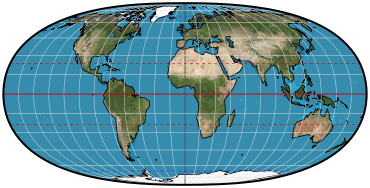## Directory of Map Projections

What is a projection?

Previous | Next

HufnagelParameters: 0° << Ψ[max] ≤ 90°, A, B, aspect ratio

Classifications

pseudocylindric
equal-area

Graticule

Meridians: Complex curves.
Parallels: Straight parallel lines.
Poles: Points if ψ[max] = 90°; otherwise lines.
Symmetry: About the central meridian and the equator.

Scale

Depends on parameterization.

Distortion

Depends on parameterization.

Parameters

ψ[max], which controls the length of the pole line. ψ[max] = 90° results in each pole being a point.
A: A configuration parameter, the effect of which depends on interaction with the other parameters. Many combinations are not allowed.
B: A configuration parameter, the effect of which depends on interaction with the other parameters. Many combinations are not allowed.
Aspect ratio: The height to width ratio desired.

Similar projections

Existing projections that Hufnagel can be parameterized to realize:.
Mollweide (= Hufnagel I): ψ[max] = 90°, A = 0, B = 0, aspect ratio = ½.
Eckert VI (approximate, = Hufnagel V): ψ[max] = 60°, A = -2/21, B = 2/21, aspect ratio = ½.
Wagner IV (= Hufnagel VI): ψ[max] = 60°, A = 0, B = 0, aspect ratio = ½.
Eckert IV (= Hufnagel VIII): ψ[max] = 45°, A = 1, B = 0, aspect ratio = ½.

Origin

Presented by Herbert Hufnagel, 1989, who devised the projection to be highly configurable while always yielding equal-area results.